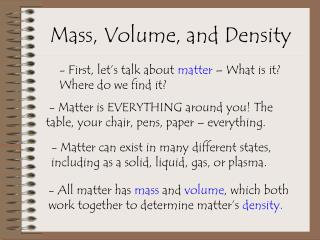DownloadDownload PresentationMass, Volume, and Density

# Mass, Volume, and Density

Télécharger la présentation## Mass, Volume, and Density

- - - - - - - - - - - - - - - - - - - - - - - - - - - E N D - - - - - - - - - - - - - - - - - - - - - - - - - - -
##### Presentation Transcript

1. Mass, Volume, and Density - First, let’s talk about matter – What is it? Where do we find it? - Matter is EVERYTHING around you! The table, your chair, pens, paper – everything. - Matter can exist in many different states, including as a solid, liquid, gas, or plasma. - All matter has mass and volume, which both work together to determine matter’s density.

2. What Holds Matter Together? • Matter can exist in many different states, including as a solid, liquid, gas, or plasma. - The low-energy molecules in a solid are tightly packed together. They don’t move very easily. If you add heat or pressure, you can make a liquid. - The medium-energy molecules in a liquid like to glide around, sliding against each other. They move in a flow. Adding heat will turn this into a gas; freezing makes a solid. - The high-energy molecules in a gas barely know each other. They move around like hyperactive bees. Adding pressure or decreasing temperature can create a liquid.

3. Mass measured in grams/kilograms, using a balance Mass is the amount of material in an object (NOT the same as weight!!) -Mass stays the same no matter what force is acting on the object. -This makes mass different from weight, which depends on both the amount of mass and the amount of gravity (measured on a scale).

4. Volume Volume is the amount of space taken up or occupied by an object. - Depending on the state (solid, liquid, gas, or plasma), volume can be measured in many ways. L x W x H L x W x H SOLID m3 LIQUID L or mL GAS L or mL PLASMA L or mL

5. A student finds a rock on the way to school.  In the lab he determines that the volume of the rock is 22.7 cm3, and the mass in 39.943 g. What is the density of the rock? Density Density is a measurement of how tightly packed matter is within a certain object. - Density is calculated by dividing mass by volume. Density = Mass (g) Volume (cm3) Density = 39.943 g 22.7 cm3 Density = 1.759 g/cm3

6. Let’s Put it All Together Mass is the amount of matter in an object or substance. - NOT the same as weight, which changes according to gravity. Volume is the amount of space an object or substance takes up. Density is the amount of matter in a certain unit of volume or space, and it is the measure of how tightly packed molecules are within a substance. - Substances of greater density will sink below those of lesser density.

7. What Does This Mean for Geology? - As you go deeper into the Earth, the density of the materials increases. The denser materials sunk down to the middle of the Earth, while the materials of lesser density float on top. - The core is composed of iron and nickel, which is the densest layer. The crust is composed mostly of silicate rock, which is the least dense. The mantle is composed of rock that has a density between that of the core and crust.